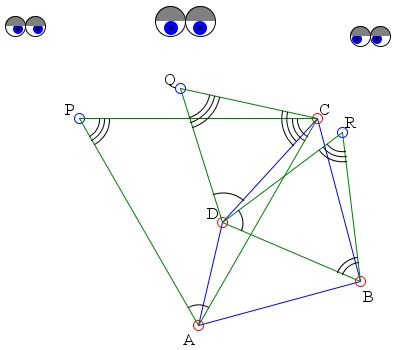## Similar Triangles on Sides and Diagonals of a QuadrilateralWhat is it about? A Mathematical Droodle

### If you are reading this, your browser is not set to run Java applets. Try IE11 or Safari and declare the site https:///www.cut-the-knot.org as trusted in the Java setup.What if applet does not run?

DiscussionThe applet may suggest the following statement [de Villiers]:

Given any quadrilateral ABCD, if similar triangles ACP, DCQ, and DBR are constructed on AC, DC, and DB, away from AB so that ∠APC = ∠ASB, where S is the intersection of AD and BC extended, then P, Q, R, S are collinear.

### If you are reading this, your browser is not set to run Java applets. Try IE11 or Safari and declare the site https:///www.cut-the-knot.org as trusted in the Java setup.What if applet does not run?

This is a generalization of a statement concerning equilic quadrilaterals; the relaxation being three-fold:

1. One does not require AD = BC;
2. One does not require ∠BAD + ∠CBA = 120°
3. The constructed triangles are similar and not necessarily equilateral.

Connect P with Q and Q with R. Construct CE parallel and equal to AD as in the applet. Let CE cuts AB in point F. Since ADCE is a parallelogram, ∠CAE = ∠DCA and ∠CFB = ∠A. In ΔCFB we therefore have

∠ECB = 180° - ∠A - ∠B = ∠APC.

From the similarity of triangles PAC and QDC, we have ∠PCA = ∠QCD. Therefore

∠PCA + ∠PCD = ∠QCD + ∠PCD.

which implies that ∠DCA = ∠QCP, and therefore,

 (1) ∠CAE = ∠QCP.

From the similarity of triangles PAC and QDC we also have PC/AC = QC/DC. But DC = AE since ADCE is a parallelogram, and therefore

 (2) PC/AC = QC/AE.

By (1) and (2), triangles AEC and CQP are similar, which implies ∠ACE = ∠CPQ. Since ∠APC = ∠ECB, we have

∠ACE + ∠ECB = ∠APC + ∠CPQ,

and so ∠ACB = ∠APQ. By constructing DG parallel and equal to CB, we can prove in a similar manner that ∠ADB = ∠QRB.

A counterclockwise rotation of size ∠PAC around A carries C to a point C' on the line through AP, and B to B'. But, since ∠ACB = ∠APQ, we have ∠AC'B' = ∠APQ and, therefore, C'B' is parallel to PQ. Thus PQ is inclined to BC at an angle of size ∠PAC. Similarly, from a clockwise rotation around B through ∠DBR that takes A to A' and D to D', we have A'D' is parallel to QR, that is, QR is inclined to AD at an angle of -∠DBR.

Since AD and BC are inclined towards each other at an angle of

180° - ∠A - ∠B = 180° - ∠PAC - ∠DBR,

rotating BC through ∠PAC and AD through -∠DBR (in appropriate direction), aligns B'C' and A'D' in the same direction, and we therefore have that PQ and QR also line up in the same direction, i.e., P, Q, and R are collinear.

Next construct ∠QS1D = ∠QCD with S1 on QR. Connect S1 with C. We now prove that ∠DS1C = 180° - ∠A - ∠B and that S1CD and S1DA are straight lines, hence S1 and S coincide.

From the construction we have QDCS1 is a cyclic quadrilateral. Thus ∠DS1C = ∠DQC = 180°. Also, ∠S1CD = ∠PQD.

In ΔPQC, we have

∠PQD = 180° - ∠CPQ - ∠QCP -∠DQC = ∠S1CD.

But

∠BCD = ∠DCA + ∠ACE + ∠ECB

and ∠DCA = ∠QCP, ∠ACE = ∠CPQ and ∠ECB = ∠DQC.

Therefore ∠S1CD + ∠BCD = 180° and S1CB is a straight line. Similarly we can prove that S1DA is a straight line. Therefore, indeed, S1 and S are one and the same point, namely the intersection of AD and BC. (Note that if S falls on QP, we simply ∠QS1C = ∠QDC and show in the same manner that S1 and S coincide.)

### References

1. M. de Villiers, The Role of Proof in Investigative, Computer-based Geometry: Some Personal Reflections, in Geometry Turned On, MAA Notes 41, 1997, pp. 15-24•